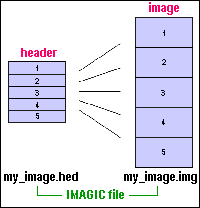## IMAGIC Formats and Conventions

IMAGIC image
Image density file
PLT file
Classification (CLS) file
Co-ordinate system
Euler angles

### IMAGIC Image

 An IMAGIC image actually consists of two files: a header file with the extension ".hed", which contains information for each image an image file with extension ".img", which contains the image densities. Note: One never has to specify the extensions ".hed" or ".img". Within IMAGIC the image name is always the one without extension.The header file has the extension ".hed" and contains information for each image

The header file contains NBLOCKS (defined in IDAT1(4)) records per image stored. The first header record for each image consists of 256 REAL/float (called DAT1) or INTEGER/int values (called IDAT1). If NBLOCK is > 1 there are additional (NBLOCKS-1) header records (each of length 256 REAL/float), which are used by some IMAGIC commands to store specific data.

The values that must be set are shown with a blue background.

### IMAGIC image density file format

The image density file has the extension ".img" and contains the image densities. This file contains only the raw data.

The raw sata can be stored in the following internal IMAGIC formats (see header index DAT1(15) - TYPE):
DBLE : Each image pixel is represented by a 64-bit real/float number
REAL : Each image pixel is represented by a 32-bit real/float number
LRGE : Each image pixel is represented by a 64-bit (signed) integer number
LONG : Each image pixel is represented by a 32-bit (signed) integer number
INTG : Each image pixel is represented by a 16-bit (signed) integer number
PACK : Each image pixel is represented by one (unsigned) byte number
COMP : Each complex image pixel is represented by 2 REAL values

The first pixel stored is the upper left one. The data is stored line by line, section by section, volume by volume. Please also refer to the definition of the IMAGIC coordinate system.

### IMAGIC PLT file formats

An IMAGIC PLT file (extension: .plt) is an ASCII format text file to store co-ordinates, euler angles, data... It can contain maximal five values per line which have to be seperated by blanks (or a comma).

PLT plot (graphics) file:
1st value: value along horizontal axis, 2nd value: value along vertical axis, 3rd value: number of curve. The first three lines for every curve can specify the title and the descriptions of the two axis.

PLT co-ordinates file:
1st value: X co-ordinate, 2nd value: Y co-ordinate, 3rd value: location number

PLT Euler angle file:
1st value: alpha, 2nd value: beta, 3rd value: gamma

PLT location number file:
(Only) one location number per line

### IMAGIC classification (CLS) file format

An IMAGIC classification CLS file (extension: .cls) is a data file an ASCII format, which contains the members belonging to the classes of an MSA classification. The information is stored class by class. For every class the following values are stored:
a) 1st line: class number, number of class members, overall class quality
b) next lines: location numbers of the class members (16 values per line)

The class members are sorted by overall quality.

### IMAGIC coordinate system

The IMAGIC coordinate system is defined as follows:

The IMAGIC coordinate system is a right-handed system with its (1,1) origin in the top-left corner of the image. The length of the lines is NY and the number of lines is NX:The centre of the image, for almost all operations on the image, is given by:

( IXMID , IYMID ) = ( NX/2 + 1 , NY/2 + 1 ).

This point is also the default centre of rotational symmetric masks and the like.

In three dimensions:### IMAGIC Euler angles

In IMAGIC the Euler angles alpha, beta, gamma are defined as three subsequent rotations. The first rotation angle ALPHA describes a rotation around the Z-axis. We then rotate along the new Y-axis over the angle BETA. Finally, the rotation GAMMY is a rotation around the new Z-axis. All rotations are counter clockwise.

Note that these are Euler angles as seen from the object.

Normally a user does not think in this way but tries to imagine how the particle would look like "in his hands":a) look at the particle along the Z-axis ("north pole")
b) rotate the particle clockwise by GAMMA
c) rotate the particle into the plane clockwise by BETA
d) rotate the particle clockwise by ALPHA